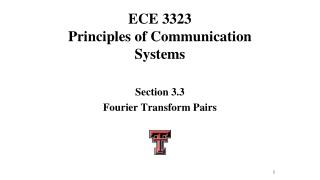Download PresentationECE 3323 Principles of Communication Systems

Loading in 2 Seconds...

# ECE 3323 Principles of Communication Systems - PowerPoint PPT Presentation

ECE 3323 Principles of Communication Systems. Section 3.3 Fourier Transform Pairs. 1. Fourier Transform of a Constant. Fourier Transform of a Constant. Fourier Transform of a Constant. 2. Fourier Transform of a Unit Impulse. Fourier Transform of a Unit Impulse.I am the owner, or an agent authorized to act on behalf of the owner, of the copyrighted work described.
Download Presentation## ECE 3323 Principles of Communication Systems

An Image/Link below is provided (as is) to download presentation

Download Policy: Content on the Website is provided to you AS IS for your information and personal use and may not be sold / licensed / shared on other websites without getting consent from its author.While downloading, if for some reason you are not able to download a presentation, the publisher may have deleted the file from their server.

- - - - - - - - - - - - - - - - - - - - - - - - - - E N D - - - - - - - - - - - - - - - - - - - - - - - - - -
Presentation Transcript
1. ECE 3323Principles of Communication Systems Section 3.3 Fourier Transform Pairs

2. 1. Fourier Transform of a Constant

3. Fourier Transform of a Constant

4. Fourier Transform of a Constant

5. 2. Fourier Transform of a Unit Impulse

6. Fourier Transform of a Unit Impulse

7. Fourier Transform of a Unit Impulse

8. 1 . . . . . . 0 -5 -4 -3 -2 -1 0 1 2 3 4 5 t 3. Fourier Transform of The Sampling Function

9. Fourier Transform of The Sampling Function

10. Fourier Transform of The Sampling Function

11. Fourier Transform of The Sampling Function

12. 1 . . . . . . 0 -5 -4 -3 -2 -1 0 1 2 3 4 5 t 1 . . . . . . 0 -5 -4 -3 -2 -1 0 1 2 3 4 5 f Fourier Transform of The Sampling Function

13. 1 . . . . . . -3 T -2 T -1 T 0 T 2 T 3 T t s s s s s s Fourier Transform of The Sampling Function

14. Fourier Transform of The Sampling Function

15. Fourier Transform of The Sampling Function

16. 1 . . . . . . -3 T -2 T -1 T 0 T 2 T 3 T t s s s s s s f s . . . . . . f -3 f -2 f -1 f 0 f 2 f 3 f s s s s s s Fourier Transform of The Sampling Function

17. 4. Fourier Transform of rect(t)

18. Fourier Transform of rect(t)

19. Fourier Transform of rect(t)

20. Fourier Transform of rect(t)

21. Fourier Transform of rect(t)

22. 5. Fourier Transform of tri(t)

23. Fourier Transform of tri(t)

24. Fourier Transform of tri(t)

25. Fourier Transform of tri(t)

26. Fourier Transform of tri(t)

27. Fourier Transform of tri(t)

28. Fourier Transform of tri(t)

29. Fourier Transform of tri(t)

30. Fourier Transform of tri(t)

31. Fourier Transform of tri(t)

32. 6. Fourier Transform of exp(– t)u(t)

33. Fourier Transform of exp(– t)u(t)

34. Fourier Transform of exp(– t)u(t)

35. Fourier Transform of exp(– t)u(t)

36. Fourier Transform of exp(– t)u(t)

37. 7. Fourier Transform of exp( j2π f0 t)

38. Fourier Transform of exp( j2π f0 t)

39. Fourier Transform of exp( j2π f0 t)

40. Fourier Transform of exp( j2π f0 t)

41. Fourier Transform of exp( j2π f0 t)

42. Fourier Transform of exp( j2π f0 t)

43. Fourier Transform of exp( j2π f0 t)

44. 8. Fourier Transform of cos(2π f0 t)

45. Fourier Transform of cos(2π f0 t)

46. Fourier Transform of cos(2π f0 t)

47. Fourier Transform of cos(2π f0 t)

48. Fourier Transform of cos(2π f0 t)